## Table of ContentOpen Access

ARTICLE

# Buoyancy Effects in the Peristaltic Flow of a Prandtl-Eyring Nanofluid with Slip Boundaries

Hina Zahir*

Department of Mathematics, Shaheed Benazir Bhutto Women University, Peshawar, 25000, Pakistan

* Corresponding Author: Hina Zahir. Email:(This article belongs to this Special Issue: Electro- magnetohydrodynamic Nanoliquid Flow and Heat Transfer)

Fluid Dynamics & Materials Processing 2023, 19(6), 1507-1519. https://doi.org/10.32604/fdmp.2023.022520

## Abstract

The interaction of nanoparticles with a peristaltic flow is analyzed considering a Prandtl-Eyring fluid under various conditions, such as the presence of a heat source/sink and slip effects in channels with a curvature. This problem has extensive background links with various fields in medical science such as chemotherapy and more in general nanotechnology. A similarity transformation is used to turn the original balance equations into a set of ordinary differential equations, which are then integrated numerically. The investigation reveals that nanofluids have valuable thermal capabilitises.

## Keywords

1  Introduction

It is a fact that peristalsis appears in the stomach, esophagus, and intestines. Movement of the bolus into the digestive tract, transport of ovum, chyme, and spermatozoa in the reproductive tract represent peristaltic motion. Owing to its significance, several investigators have considered this phenomenon in articles. Nadeem et al.  analyzed peristalsis with sinusoidal walls in a duct. Further Ali et al.  discussed the thermodynamics of nonlinear convection in peristalsis. Gangavathi et al.  studied the peristaltic pumping in a porous medium. Narla et al.  investigated wave propagation by considering the peristaltic motion of spermatozoa. In straight microchannel, Waheed et al.  indicates heat stream in electromotive biofluid flow which involves the peristalsis. The peristaltic flow of viscoelastic fluid with the Hartmann boundary layer effect is investigated by Ali et al. . Akram and Rashid discussed the peristaltic flow of Rabinowitsch and Williamson fluid respectively (see [7,8]). An experimental approach is discussed by Mirzaei et al.  in dialysis machines of peristaltic slug flows. Saleem et al.  and Akram et al.  also studied peristaltic flow in different channels.

In the heat transfer process, when free and forced convection both takes part, it is then known as mixed convection. In several technological and scientific fields such as biology, geology chemical processes, astrophysics, etc., this effect is utilized. The importance of peristalsis with convective heat exchange cannot be underestimated as it has several examples, i.e., in translocation of water in tall trees, solar ponds, dynamic of lakes, diffusion of nutrients out of blood, lubrication and drying technologies, oxygenation, hemodialysis, and nuclear reactors. Also due to, its vast usage different researchers work on it. Akbar et al.  found irreversibility rate with taking mixed convective effect. Mixed Marangoni flow of copper hybrid and aluminum oxide nanofluid is discussed by Li et al. . Song et al. also discussed mixed convection in rotating and inclined channels respectively (see [14,15]).

Nanomaterial is a mixture of nanoparticles and base fluid. Nanomaterials hold great significance in textile fiber production, cooling of fluid in nuclear reactors, reservoirs of petroleum, cancer therapy, and surgical processes. This phenomenon was first studied by Choi et al. . Hayat et al.  considered this phenomenon for the peristaltic flow of fourth-grade fluid. Bashir et al.  considered magnetic flux and Fourier/Ficks theories on effects in the peristaltic motion of Carreau-Yasuda nanofluid. Zahid et al.  showed informative results by taking hybrid nanofluid with entropy effect. Thermal analysis in curved microchannels via nanofluids is investigated by Akram et al. . Further research in this context can be seen through refs. .

The present investigation aims to venture further into the regime of peristaltic transport of nanofluids with base material satisfying rheological characteristics. A curved channel curve is considered for the description of a more realistic situation. Peristaltic transport of Prandtl-Eyring nanofluid (as this fluid consideration is significant for various applications in biomedicine and engineering) in a curved channel is examined subject to mixed convection, radiation, and heat generation/absorption. Unlike the traditional attempts, the partial slip features for temperature, velocity, and concentration are implemented. Governing flow problem is simulated numerically. The physical interpretation is arranged and some conclusions are pointed out.

2  Formulation

Here peristaltic transport of Prandtl--Eyring nanofluid in the curved channel is examined. The coordinate system (r, x) is chosen. Here r is along the radial direction and x normal to r (see Fig. 1). Brownian motion and thermophoresis characterize the nanofluid. Mixed convection is considered. Flow analysis is carried out when no-slip conditions for velocity, emperature, and concentration remain no longer valid.

r=± η(x, t)=±[d+asin2πλ(xct)], (1)

where λ , a, t, denote the wavelength, amplitude, and time. The boundary walls are represented by η and η, respectively.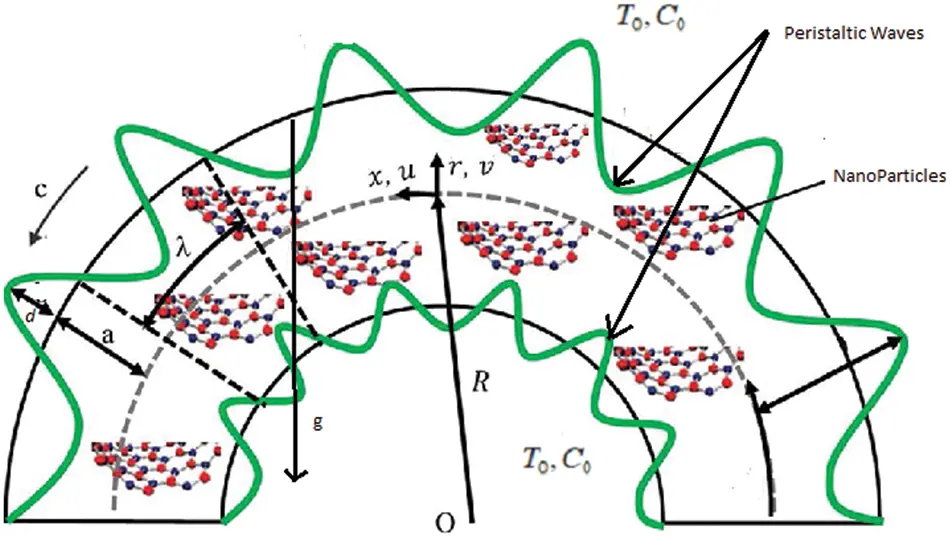Figure 1: Schematic representation of the electroosmotically modulated peristaltic propulsion in the curved microchannel

Governing equations can be formulated under the considered assumptions (see [5,8,10]):

Continuity Equation:

vr+Rr+Rux+vr+R=0. (2)

Momentum Equations:

ρf(vt+vvr+Rr+Ruvxu2r+R)=Pr+1r+Rr[(r+R)Srr]+Rr+RSrxxSxxr+R,  (3)

ρf(ut+vur+Rr+Ruux+uvr+R)=Rr+RPx+1(r+R)2r[(r+R)2Sxr]+Rr+RSxxx+ρgβT(TT0)+ρgβC(CC0). (4)

Heat Equation:

(cρ)f(Tt+vTr+Rr+RuTx)=k1(2Tr2+1r+RTr+Rr+R2Tx2)+(cρ)pDTTm[(Rr+R)22Tx2+2Tr2]+(cρ)pDB[(Rr+R)2TxCx+TrCr]+Q0. (5)

Concentration Equation:

(Ct+vCr+Rr+RuCx)=DB(2Cr2+1r+RCr+Rr+R2Cx2)+DTTm[2Tr2+1r+RTr+Rr+R2Tx2]. (6)

Prandtl fluid’s stress tensor is given by

S=1γ˙Asinh1(γ˙C)A1, (7)

γ˙=Π2,

Π=trA12,

A1=V+(V)T,

sinh1(γ˙C)γ˙C16(γ˙C)3,  (γ˙C)51. (8)

In the above equations velocity field is represented by V=(v(r, x, t), u(r, x, t), 0) , material derivative by ddt=t+vr+Rur+Rx, fluid density by ρf , gravitational acceleration by g, thermal and concentration expansion by βT and βC , thermal and concentration at boundaries by ( T0 , T ) and ( C0 , C ), Brownian and thermophoresis parameters by DB and DT , mean temperature by Tm , heat source/sink by Q0 , thermal conductivity by k1 , fluid materials by A and C, and first Rivlin Erickson tensor by A1 .

Velocity, temperature, and concentration slip boundary conditions are given by

u+α1Srx=0 at r=+η,  uα1Srx=0 at r=+η,  (9)

T+α2Tr=T0 at r=+η, Tα2Tr=T0 at r=η,  (10)

C+α3Cr=C0 at r=+η, Cα3Cr=C0 at r=η (11)

Compliant Wall Condition:

Rr+R(τ3x3+m13xt2+d2xt)η=ρf(ut+vur+Rr+Ruux+uvr+R)+1(r+R)2r[(r+R)2Sxr]+Rr+RSxxx+ρgβT(TT0)+ρgβC(CC0)       at    r=±η. (12)

Here boundary slip, elastic parameter, damping, and mass/area are denoted by αi(i=13) , τ , d , and m1 , respectively. If ψ represents stream function then

u=ψr,  v=Rr+Rψx. (13)

We use

ψ=ψcd, x=xλ,  r=rd,  t=ctλ, u=uc, α=Aμc, β=αc2C2d12, v=vc, η=ηd, θ=TT0T0, φ=CC0C0, p=d2pμcλ, Qr=ρgβCd2μcC0, u=ψr, v=δkr+kψx, Gr=ρgβTd2μcT0, k=Rd, S=dμcS, δ=dλ, S=Q0d2k1, Pr=μcfk1, Nb=τDBC0k1, Nt=τDTT0k1Tm, E1=τd3λ3μc, E2=m1cd3λ3μ, E3=d3dλ2μ, ε=ad. (14)

And applying long wavelength and small Reynolds number approximations one obtains

pr=0,  (15)

kr+kpx+1(r+k)2r[(r+k)2Sxr]+Grθ+Qrφ=0,  (16)

(2θr2+1r+kθr)+PrNb(θrφr)+PrNt(2θr2)+S=0,  (17)

(2φr2+1r+kφr)+NtNb(2θr2+1r+kθr)=0. (18)

Here Pr represents the Prandtl number, Gr and Qr are Grashof numbers for local temperature and local nanoparticles mass transfer. Nt and Nb the thermophoresis and Brownian motion parameters and E1 , E2, E3 the wall elasticity parameters, respectively.

Non-dimensional conditions are

η=1+εsin2π(xt),  (19)

ψr+α1Srx=0 at r=+η,

ψrα1Srx=0 at r=η,

θ+α2θr=0 at r=+η,  (20)

θα2θr=0 at r=η,

φ+α3φr=0 at r=+η,

φα3φr=0 at r=η.

kr+k(E13x3+E23xt2+E32xt)η=1(r+k)2r[(r+k)2Sxr]+Grθ+Qrφ at r=±η. (21)

Non-dimensional stress component is

Srx=α(ψrr+ψrr+k)β6(ψrr+ψrr+k)3,  (22)

in which α and β are Prandtl-Eyring parameters.

The rate of heat transfer coefficient Z can be defined as

Z=ηx|θr|r=η. (23)

3  Solution Methodology

In the above-mentioned descriptions, the system of non-linear coupled equations is derived whose exact solutions are difficult to obtain by solving explicitly. However, during the last few decades, the advancement of techniques come up with more effective ways to solve non-linear problems. Thus present problem can be tackled by the NDSolve routine of Mathematica which is a built-in solver of the mathematical software. Such a technique is useful for choosing a suitable algorithm automatically and it will track errors from provided maximum to minimum range. Further, it provides graphical illustrations directly and intricated solution expressions are avoided.

4  Results and Discussion

The behavior of the obtained solutions is visualized by plotting the graphs for different flow properties such as velocity, thermal distribution, heat transfer coefficient, etc., in the given section.

4.1 Velocity

In this subsection graphs of velocity for various parameters of interest are plotted. The influence of the curvature parameter on the velocity profile u is shown in Fig. 2. For larger k the velocity has dual behavior. In the lower half of the channel u decays while velocity is increased in the upper half of the channel. Because of the non-symmetry of velocity about the center line in a curved channel. In Fig. 3 increasing behavior of fluid velocity is observed for a rise in the wall parameters E1 and E2 which is due to the fact that the more elasticity in the walls offers less resistance to flow. However, the opposite effect is noticed for E3 , similar results are found by Akram et al. . Impacts of Gr and Qr on velocity are seen in Figs. 4 and 5. With the increase in Gr the velocity enhances whereas for larger Qr opposite behavior is noticed. It is because of the reduction in viscosity. These parameters arising due to mixed convection are found substantially useful in heating or cooling of channel walls with small separation and in the case of laminar flow to dissipate energy more actively than forced convection. Akbar et al.  also showed similar results. Figs. 6 and 7 are portrayed to see the behavior of α and β on u. Velocity becomes more for larger α and β . In fact, fluid viscosity decays which is responsible for an increase in u. Fig. 8 shows increasing behavior for slip parameter α1 on velocity (same as ). Since kinetic energy enhances with a deviation of fluid particles along slip wall and thus u increases.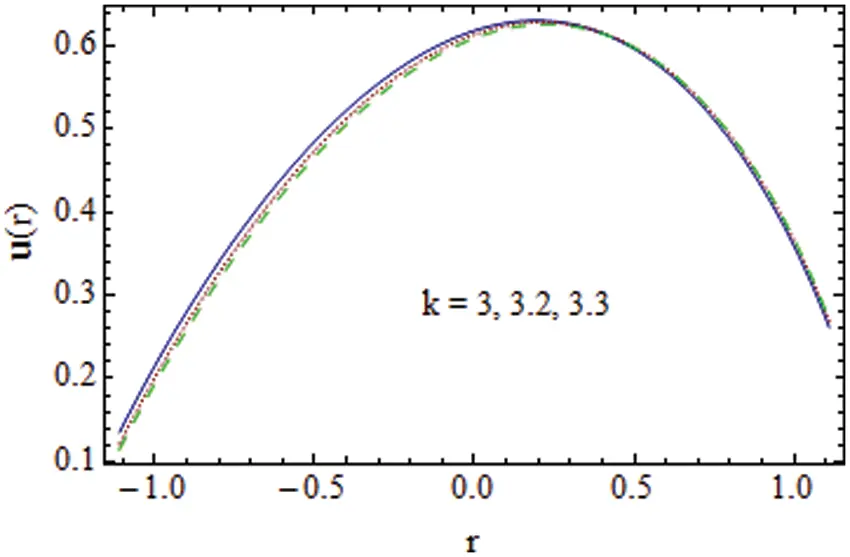Figure 2: k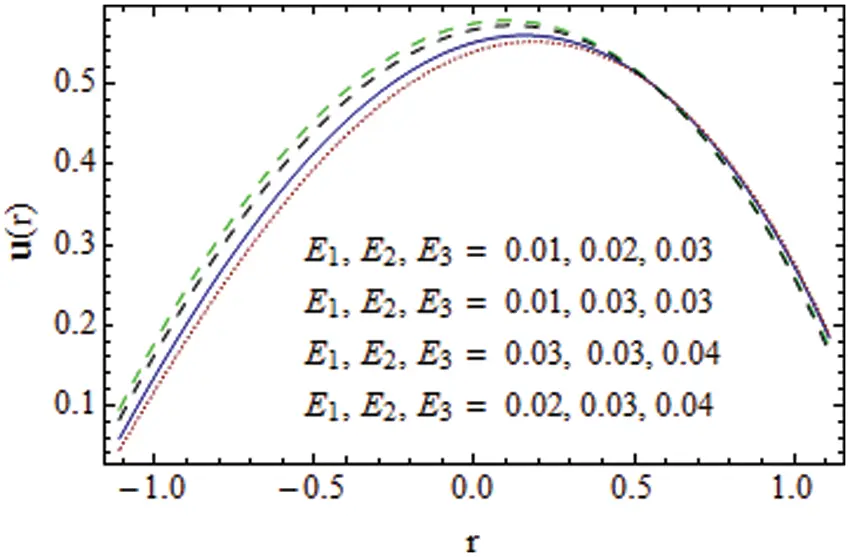Figure 3: E1, E2, E3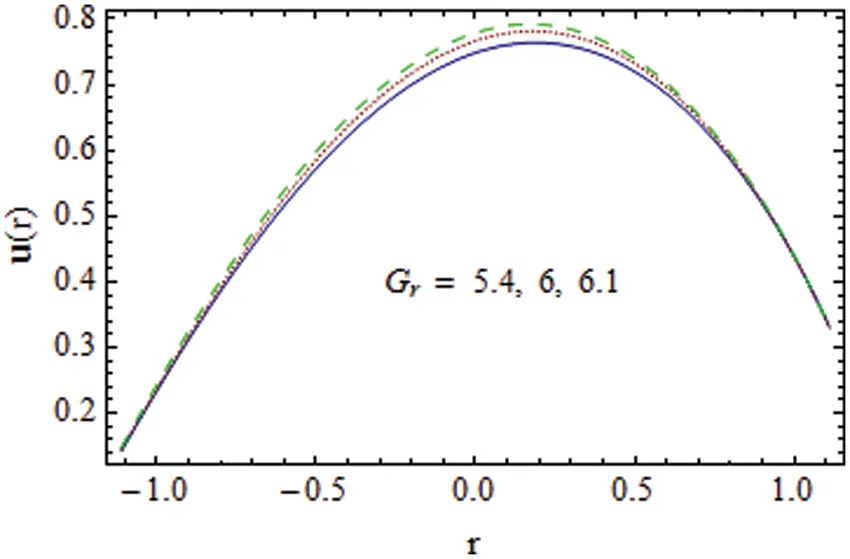Figure 4: Gr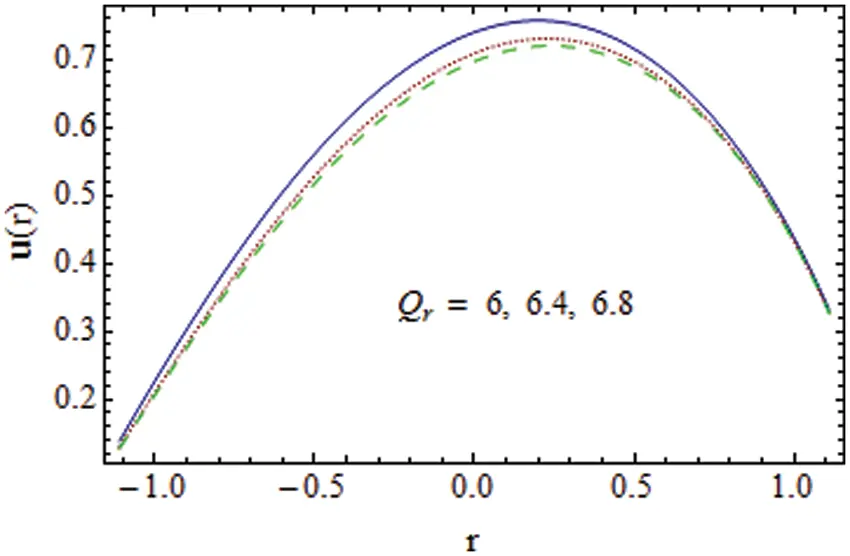Figure 5: Qr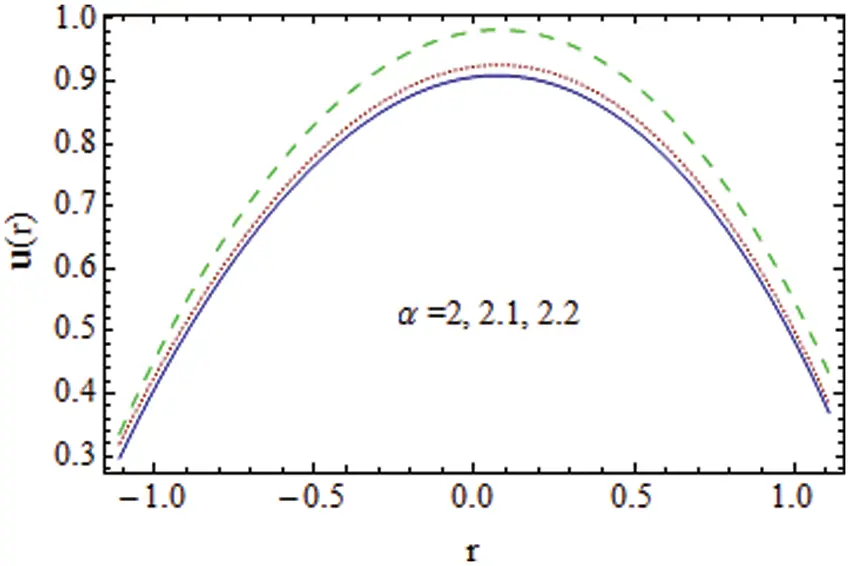Figure 6: α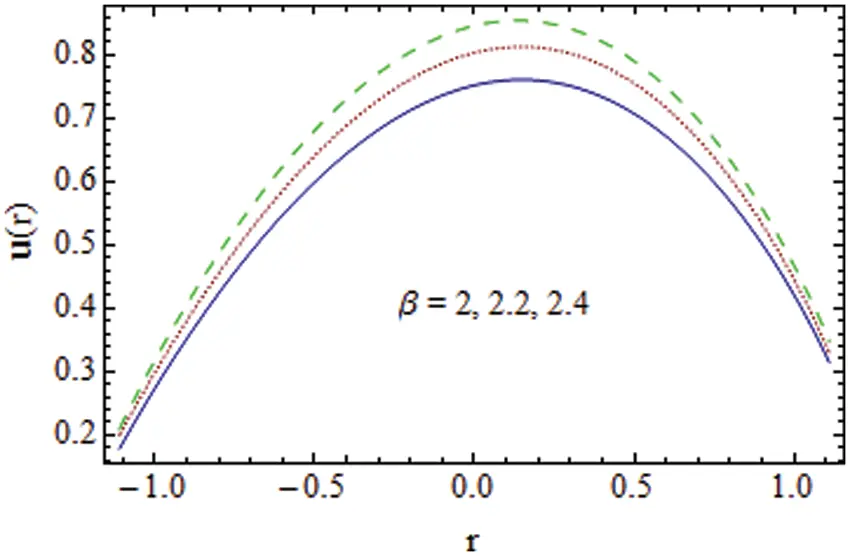Figure 7: β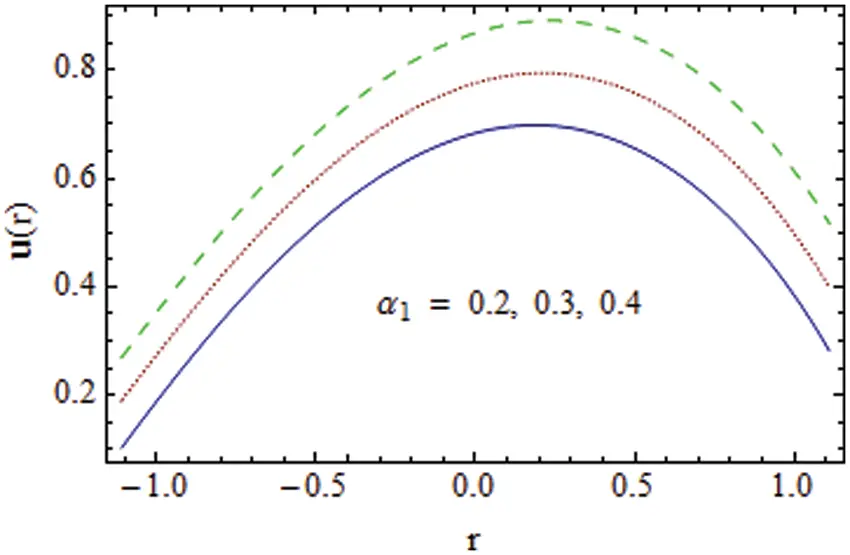Figure 8: α1 on velocity u

4.2 Thermal Field

Increasing behavior of thermal slip α2 on θ is observed in Fig. 9. It is because more heat transfer occurs with a large temperature difference between walls and fluid from one point to another. Larger values of the Prandtl number give decreasing behavior of temperature (see Fig. 10). It is because of the reduction in thermal conductivity. The opposite outcome of θ is seen for increasing Nb and Nt . For higher values of Nt, θ decays whereas it grows for Nb (see Figs. 11 and 12). By increasing Nt , thermophoresis forces become strengthened and these forces tend to shift nanoparticles from the hotter region towards a cooler region. Hence thickness of the boundary enlarges and θ boosts. Such results in a limiting sense are reported by Hayat et al. . The thermal field of fluid enhances for S as we move from negative to positive values and consequently θ rises. This impression is quite obvious since enhancement in S corresponds to the increasing strength of the heat source parameter which tends to raise the nanofluid temperature (see Fig. 13). Since the mass characterizing E1 and elasticity E2 parameters increase the speed of flow so θ enhances. However, θ via E3 has a decreasing effect (see Fig. 14). The speed of the flow (cardiovascular compliance) grows as E1 and E2 elevate and particles moving with high velocities elevate the flow, whereas speed reduces for damping coefficient E3 and temperature of moving fluid. Similar results are found in .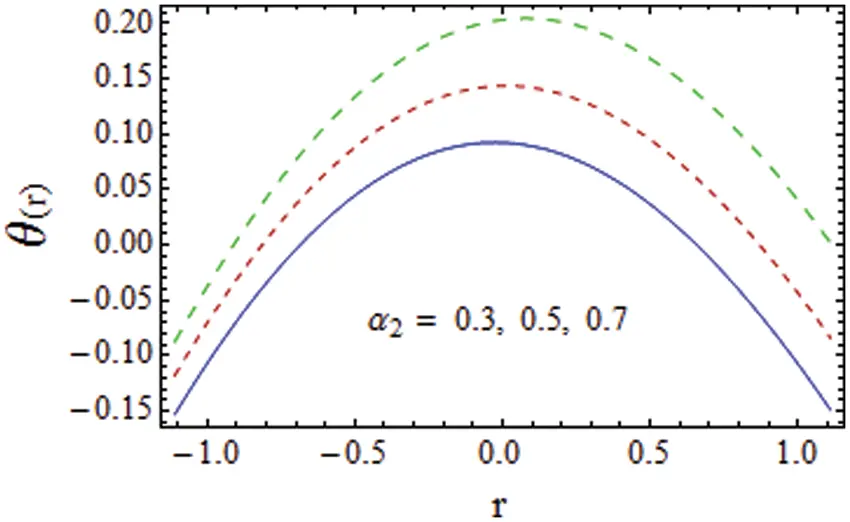Figure 9: α2Figure 10: Pr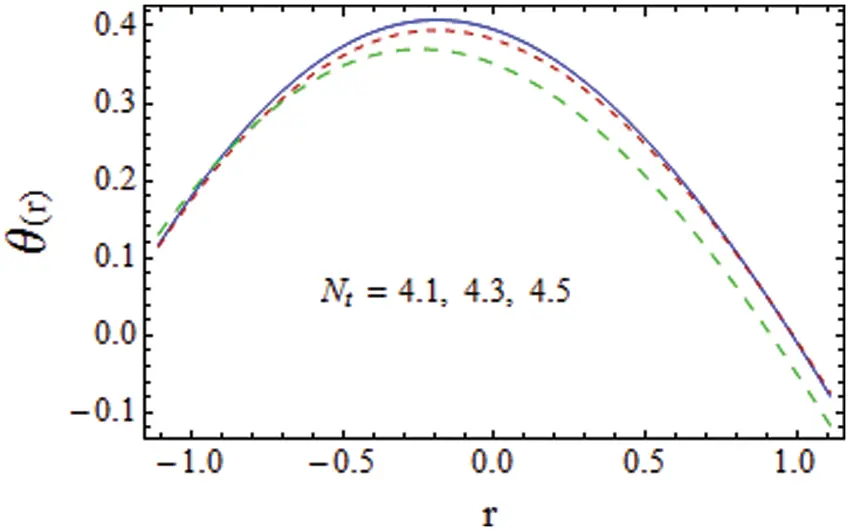Figure 11: NtFigure 12: Nb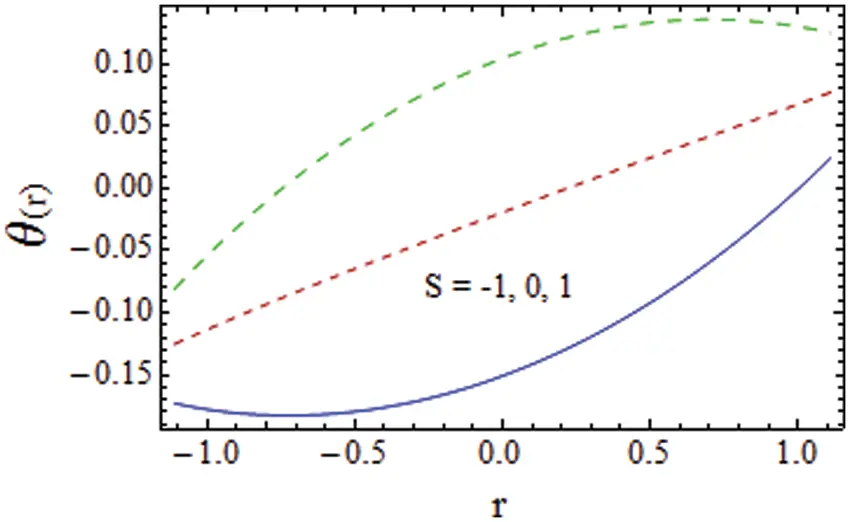Figure 13: S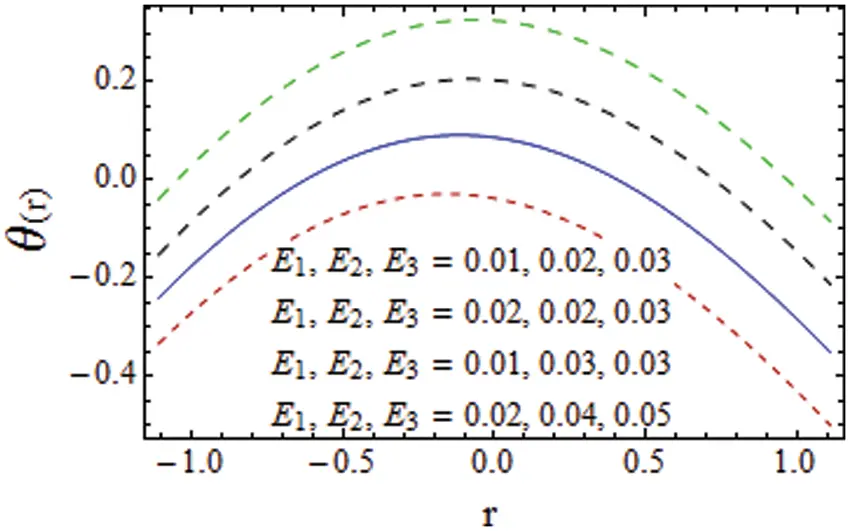Figure 14: I E1, E2, E3 on the thermal field θ

4.3 Heat Transfer Coefficient

The impact of various parameters on heat transfer coefficient Z is discussed in this section through Figs. 1517. By increasing Brownian diffusion Nb reduction in Z is noticed (see Fig. 15). Opposite result is seen for Nt in Fig. 16. Similar results are studied by Hayat et al. . Fig. 17 shows increasing behavior of Z for S the same reason as discussed in the temperature graphs.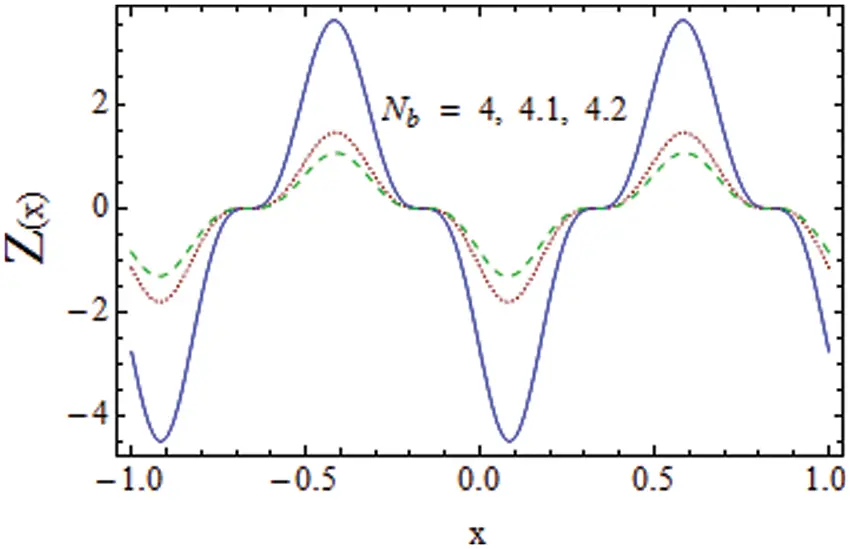Figure 15: Nb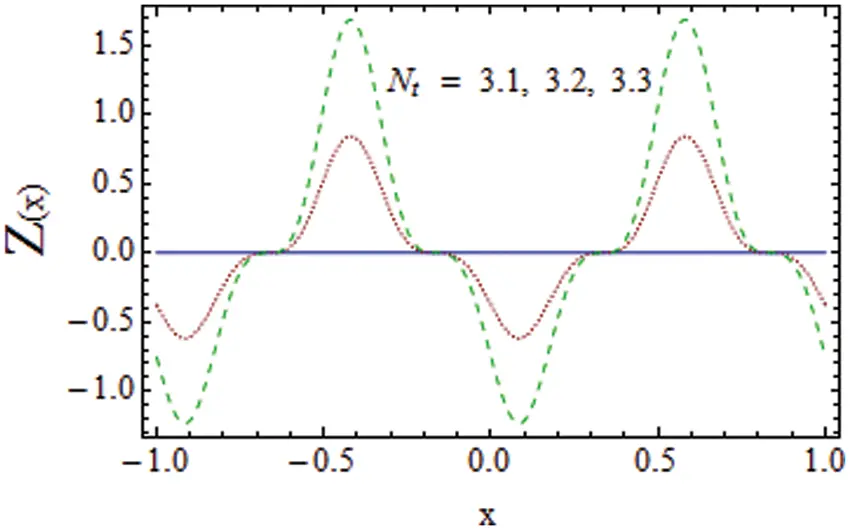Figure 16: Nt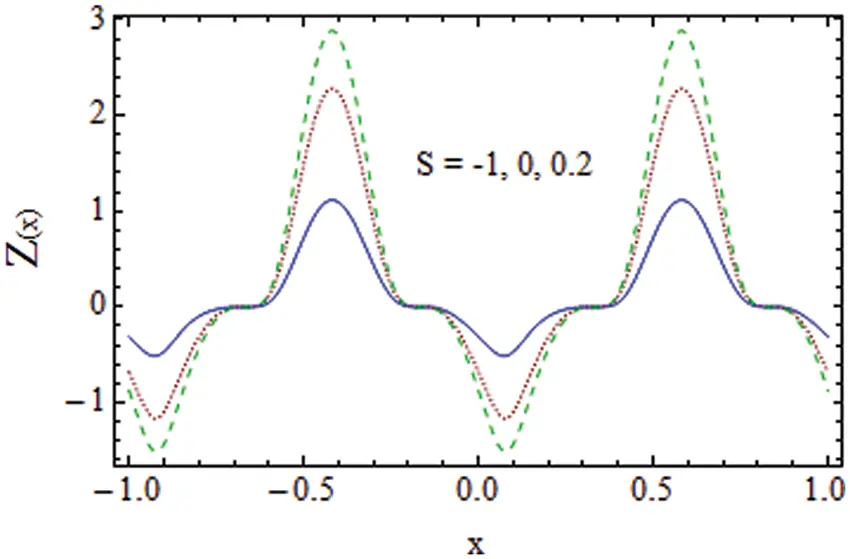Figure 17: S on heat transfer coefficient Z

4.4 Concentration

Effects of involved parameters on φ are discussed in this subsection. Increasing behavior of φ is observed for α while opposite behavior is noticed via β (see Figs. 18 and 19). As the density of nanoparticles becomes higher for increasing Nb , therefore, a rise in concentration occurs (see Fig. 20). Opposite outcome of φ for larger Nt is shown in Fig. 21. Obviously φ decays for increasing Nt. Fig. 22 is portrayed to see the behavior of α3 on concentration. Due to slip effects, the disturbance on the fluid caused by the wall is less, and less mass transfer occurs.Figure 18: Impact of α on φ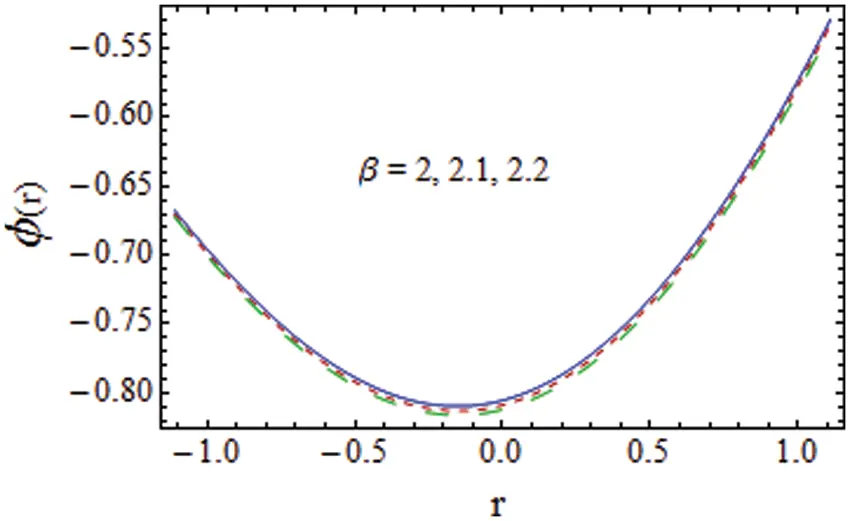Figure 19: Impact of β on φ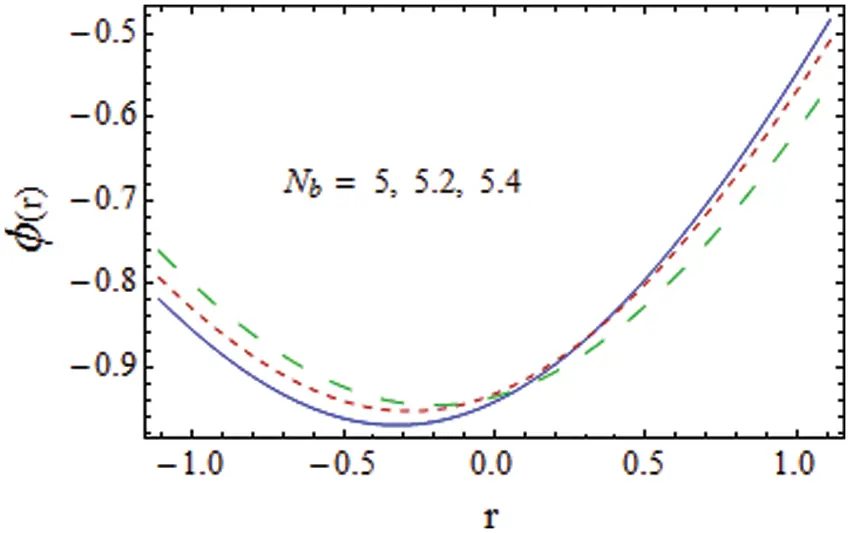Figure 20: Impact of Nb on φ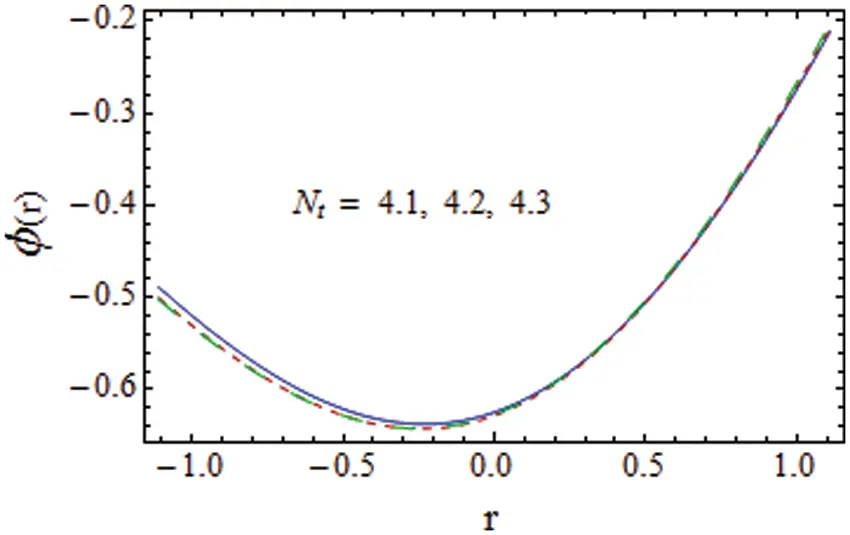Figure 21: Impact of Nt on φ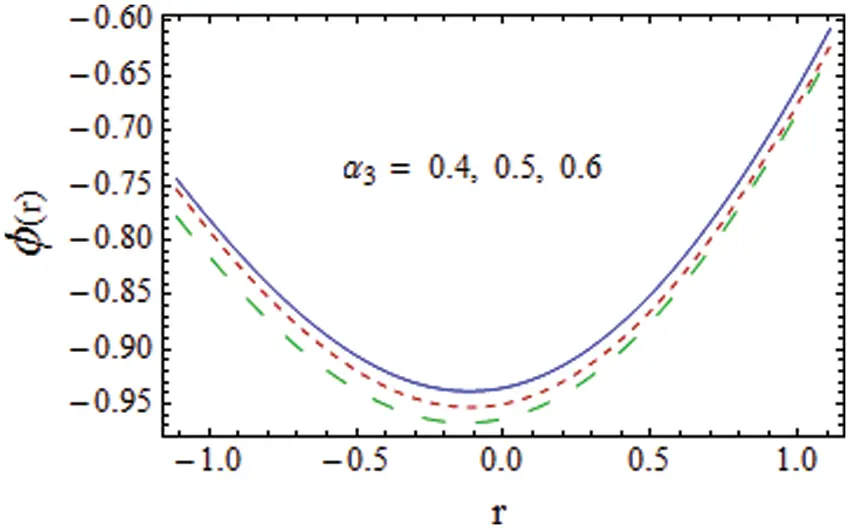Figure 22: Impact of α3 on φ

4.5 Trapping

Fig. 23 examines the impact of k on temperature. Here bolus size decays and the number of circulation increases for larger k. There is an increase in bolus size via Gr (see Fig. 24). Qr effects on bolus size is opposite to that of Gr (see Fig. 25). Figs. 26 and 27 account that number of circulation and size of bolus remains unchanged when Nt and Nb are enhanced.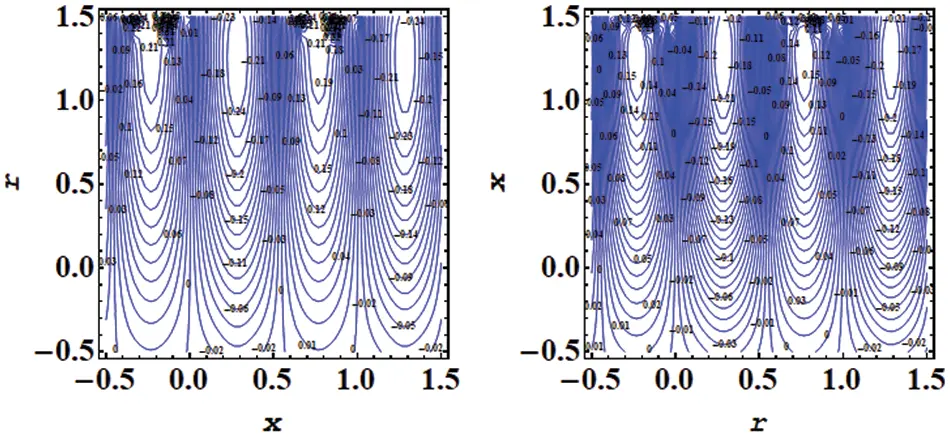Figure 23: Impact of streamlines for various values of k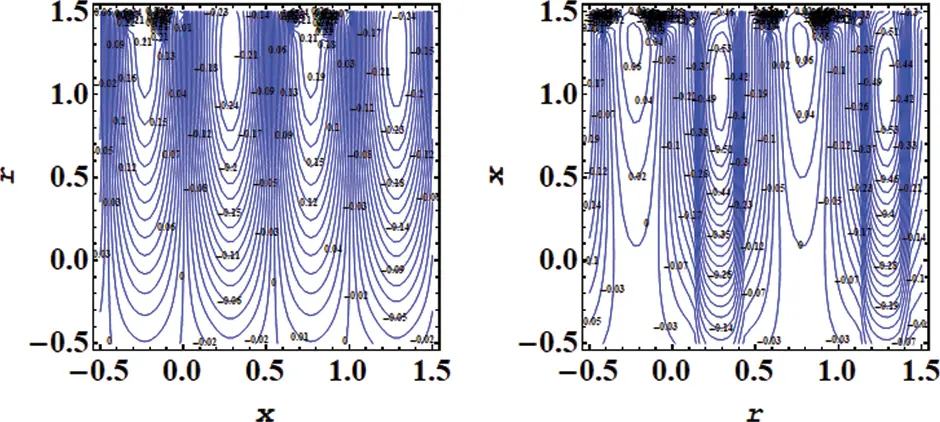Figure 24: Impact of streamlines for various values of GrFigure 25: Impact of streamlines for various values of Qr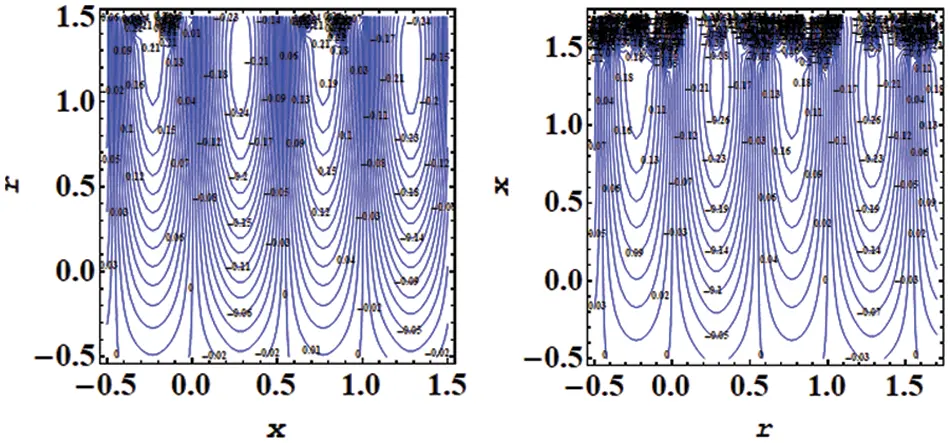Figure 26: Impact of streamlines for various values of Nt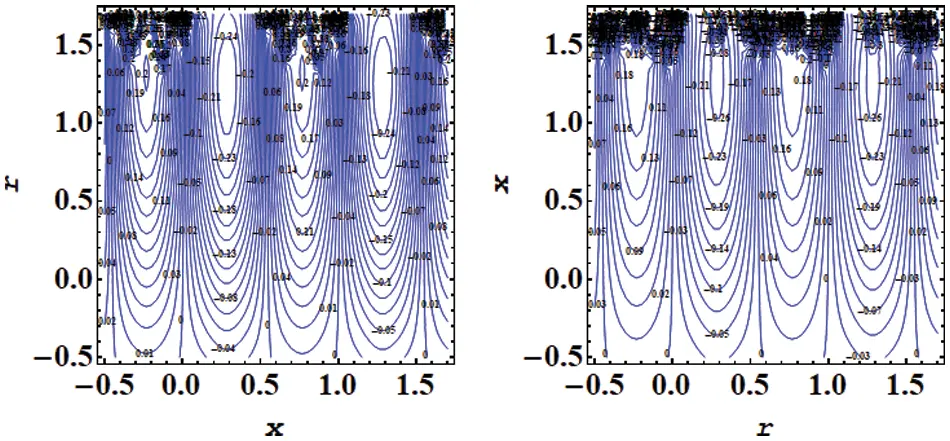Figure 27: Impact of streamlines for various values of Nb

5  Conclusions

Here an investigation is being carried out on the peristaltic flow of Prandtl fluid in the presence of mixed convection across a curved channel and the influence of heat source/sink is also accounted. The following key features of the analysis are worth mentioning:

•   Fluid parameters tend to increase velocity.

•   The opposite behavior is noticed for Grashof numbers Qr and Gr towards velocity.

•   Similar behavior is observed for thermophoresis and Brownian motion on temperature and concentration.

•   Velocity and temperature show enhancement for wall parameters whereas temperature decays for damping parameters.

•   For a larger heat source/sink, the temperature and heat transfer coefficient rises.

•   Heat transfer for thermophoresis and Brownian motion parameters has an opposite response.

•   Outcomes of slip parameters on velocity and temperature are similar.

Funding Statement: The author received no specific funding for this study.

Conflicts of Interest: The author declares that they have no conflicts of interest to report regarding the present study.

## References

1. Nadeem, S., Akhtar, S., Alharbi, F. M., Saleem, S., & Issakhov, A. (2022). Analysis of heat and mass transfer on the peristaltic flow in a duct with sinusoidal walls: Exact solutions of coupled PDEs. Alexandria Engineering Journal, 61(5), 4107-4117. [Google Scholar] [CrossRef]
2. Ali, Z., Qasim, M., & Ashraf, M. U. (2021). Thermodynamic analysis of nonlinear convection in peristaltic flow. International Communication in Heat and Mass Transfer, 129, 105686. [Google Scholar] [CrossRef]
3. Gangavathi, P., Jyothi, S., Subba Reddy, M. V., Yogeswara Reddy, P. (2021). Slip and hall effects on the peristaltic flow of a jeffrey fluid through a porous medium in an inclined channel. Materialstoday Procedures. DOI 10.1016/j.matpr.2021.05.696. [CrossRef]
4. Narla, V. K., & Tripathi, D. (2020). Entropy and energy analysis on peristaltic pumping in a curved narrow channel. Heat Transfer, 49(6), 3357-3373. [Google Scholar] [CrossRef]
5. Waheed, S., Lu, D. C., & Tripathi, D. (2021). Heat stream in electroosmotic bio-fluid flow in straight microchannel via peristalsis. International Communications in Heat and Mass Transfer, 123, 105180. [Google Scholar] [CrossRef]
6. Ali, A., Awais, M., Al-Zubaidi, A., Saleem, S., & Marwat, D. N. K. (2022). Hartmann boundary layer in peristaltic flow for viscoelastic fluid: Existence. Ain Shams Engineering Journal, 13(2), 101555. [Google Scholar] [CrossRef]
7. Akram, J., Akbar, N. S., & Tripathi, D. (2021). Entropy generation in electroosmotically aided peristaltic pumping of MoS rabinowitsch nanofluid. Fluid Dynamics Research, 54(1), 015507. [Google Scholar] [CrossRef]
8. Rashid, M., Ansar, K., & Nadeem, S. (2020). Effects of induced magnetic field for peristaltic flow of williamson fluid in a curved channel. Physica A: Statistical Mechanics and its Applications, 553, 123979. [Google Scholar] [CrossRef]
9. Mirzaei, M., & Parrany, M. (2021). An experimental study on real–time analysis of two-phase peristaltic slug flows in dialysis machines. Flow Measurement and Instrumentation, 79, 101941. [Google Scholar] [CrossRef]
10. Saleem, A., Akhtar, S., Alharbi, F. M., Nadeem, S., & Ghalambaz, M. (2020). Physical aspects of peristaltic flow of hybrid nanofluid inside a curved tube having a ciliated wall. Results in Physics, 19, 103431. [Google Scholar] [CrossRef]
11. Akram, S., Saleem, N., Umair, M. Y., & Munawar, S. (2021). Impact of partial slip and lateral walls on peristaltic transport of a couple stress fluid in a rectangular duct. Science Progress, 104(2), 1-17. [Google Scholar] [CrossRef]
12. Akbar, Y., Abbasi, F. M., Zahid, U. M., & Shehzad, S. A. (2021). Effectiveness of heat and mass transfer on mixed convective peristaltic motion of nanofluid with irreversibility rate. Communications in Theoretical Physics, 73(10), 105004. [Google Scholar] [CrossRef]
13. Li, X. L., Khan, M., Punith Gowda, R. J., Ali, A., & Shahid, F. (2021). Dynamics of aluminum oxide and copper hybrid nanofluid in nonlinear mixed marangoni convective flow with entropy generation: Applications to renewable energy. Chinese Journal of Physics, 73(1), 275-287. [Google Scholar] [CrossRef]
14. Song, Y. Q., Khan, S. A., Imran, M., Waqas, H., & Khan, S. U. (2021). Applications of modified darcy law and nonlinear thermal radiation in bioconvection flow of micropolar nanofluid over an off centered rotating disk. Alexandria Engineering Journal, 60(5), 4607-4618. [Google Scholar] [CrossRef]
15. Vedavathi, N., Dharmaiah, G., Abdul Gaffar, S., & Venkatadri, K. (2021). Entropy analysis of nanofluid magnetohydrodynamic convection flow past an inclined surface: A numerical review. Heat Transfer, 50, 5996-6021. [Google Scholar] [CrossRef]
16. Choi, S. U., & Eastman, J. A. (1995). Enhancing thermal conductivity of the fluids with nanoparticles. ASME International Mechanical Engineering Congress & Expositions: Vol. 231, pp. 99-105. Argonne.
17. Hayat, T., Nisar, Z., & Alsaedi, A. ((2020)). Impacts of slip in radiative MHD peristaltic flow of fourth-grade nanomaterial with chemical reaction. International Communication in Heat and Mass Transfer, 119, 104976. [Google Scholar]
18. Bashir, S., Ramzan, M., Chung, J. D., Chu, Y. M., Kadry, S. (2021). Analyzing the impact of induced magnetic flux and fourier’s and fick’s theories on the carreau-yasuda nanofluid flow. Science Report, 9230. DOI 10.1038/s41598-021-87831-6. [CrossRef]
19. Zahid, U. M., Akbar, Y., & Abbasi, F. M. (2020). Entropy generation analysis for peristaltically driven flow of hybrid nanofluid. Chinese Journal of Physics, 67, 330-348. [Google Scholar] [CrossRef]
20. Akram, J., Akbar, N. S., & Tripathi, D. (2021). Thermal analysis on MHD flow of ethylene glycol-based BNNTs nanofluids via peristaltically induced electroosmotic pumping in a curved microchannel. Arabian Journal for Science and Engineering, 47, 7487-7503. [Google Scholar] [CrossRef]
21. Narla, V. K., Tripathi, D., & AnwarBég, O. (2020). Electro-osmotic nanofluid flow in a curved microchannel. Chinese Journal of Physics, 67, 544-558. [Google Scholar] [CrossRef]
22. Aldabesh, A., Khan, S. U., Habib, D., Waqas, H., & Tilili, A. (2020). Unsteady transient slip flow of williamson nanofluid containing gyrotactic microorganism and activation energy. Alexandria Engineering Journal, 59(6), 4315-4328. [Google Scholar] [CrossRef]
23. Ali, B., Thumma, T., Habib, D., Salamat, N., & Riaz, S. (2022). Finite element analysis on transient MHD 3D rotating flow of Maxwell and tangent hyperbolic nanofluid past a bidirectional stretching sheet with cattaneo christov heat flux model. Thermal Science and Engineering Progress, 28, 101089. [Google Scholar] [CrossRef]
24. Ali, B., Siddique, I., Ahmadian, A., Senu, N., & Ali, L. (2022). Significance of lorentz and coriolis forces on dynamics of water-based silver tiny particles via finite element simulation. Ain Shams Engineering Journal, 13(2), 101572. [Google Scholar] [CrossRef]
25. Ali, B., Siddique, I., Khan, I., Masood, B., & Hussain, S. (2021). Magnetic dipole and thermal radiation effects on hybrid base micropolar CNT flow over a stretching sheet: Finite element method approach. Results in Physics, 25, 104145. [Google Scholar] [CrossRef]
26. Tamizharasi, P., Vijayaragavan, R., & Magesh, A. (2021). Heat and mass transfer analysis of the peristaltic driven flow of a nanofluid in an asymmetric channel. PDEs Applied Mathematics, 4, 100087. [Google Scholar] [CrossRef]
27. McCash, L. B., Akhtar, S., Nadeem, S., & Saleem, S. (2021). Entropy analysis of the peristaltic flow of hybrid nanofluid inside an elliptic duct with sinusoidally advancing boundaries. Entropy, 23, 732. [Google Scholar] [CrossRef]
28. Ahmed, B., Hayat, T., Alsaedi, A., & Abbasi, F. M. (2020). Entropy generation analysis for peristaltic motion of carreau–Yasuda nanomaterial. Physica Scripta, 95(5), 055804. [Google Scholar] [CrossRef]
29. Nisar, Z., Hayat, T., Alsaedi, A., & Ahmad, B. (2022). Significance of activation energy in radiative peristaltic transport of eyring-powell nanofluid. International Communications in Heat and Mass Transfer, 116, 104655. [Google Scholar] [CrossRef]

Zahir, H. (2023). Buoyancy Effects in the Peristaltic Flow of a Prandtl-Eyring Nanofluid with Slip Boundaries. FDMP-Fluid Dynamics & Materials Processing, 19(6), 1507–1519.This work is licensed under a Creative Commons Attribution 4.0 International License , which permits unrestricted use, distribution, and reproduction in any medium, provided the original work is properly cited.

View

Like

## Related articles

• M. Ihdene, T. Ben Malek, S. Aberkane,...
• S. Slama, H. Kahalerras, B. Fersadou...